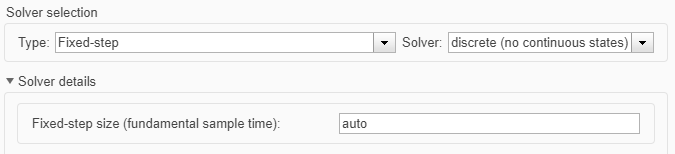## Simulation Acceleration by Using GPU Coder

You can use GPU Coder™ to speed up the execution of your Simulink® model by using NVIDIA® GPUs. If you have algorithms written in MATLAB® code, you can include the MATLAB code in a Simulink model or subsystem by using the MATLAB Function (Simulink) block. When you simulate a model that contains a MATLAB Function block, the software generates CUDA® MATLAB executable (MEX) code from the block and dynamically links the generated code to Simulink. For more information on code generation workflows supported by GPU Coder, see GPU Code Generation Workflow.

The basic steps for simulation acceleration by using GPU are:

• Create or open a model

• Configure the model for GPU acceleration by selecting the solver, language, and other GPU-specific configuration parameters

• Run the GPU accelerated model

### Example: Sobel Edge Detection

The Sobel edge detection algorithm is a popular yet simple edge detection algorithm that performs a 2-D spatial gradient operation on a grayscale image. This operation emphasizes the high spatial frequency regions which corresponds to the edges of the input image.

The Sobel edge algorithm computes the horizontal gradient (`H`) and the vertical gradient (`V`) of the input image by using two orthogonal filter kernels (`k` and `k'`). After the filtering operation, the algorithm computes the gradient magnitude and applies a threshold to find the regions of the images that are considered to be edges.

```k = single([1 2 1; 0 0 0; -1 -2 -1]); H = conv2(single(grayImage),k, 'same'); V = conv2(single(grayImage),k','same'); E = sqrt(H.*H + V.*V); edgeImage = uint8((E > threshold) * 255); ```### Create Edge Detection Model

1. Create a new Simulink model and insert two MATLAB Function blocks from the User-Defined Functions library.

2. Add a Constant (Simulink) block and set its value to `0.4`. Add a From Multimedia File (Computer Vision Toolbox) block from the Computer Vision Toolbox™ library.

3. Open the Block Parameters dialog for the From Multimedia File block and set the File name parameter to `rhinos.avi`.

Set the Image signal parameter to ```One multidimensional signal```.

4. Add two Video Viewer (Computer Vision Toolbox) blocks from the Computer Vision Toolbox library to the model.5. Double-click on one of the MATLAB Function blocks. A default function signature appears in the MATLAB Function Block Editor.

6. Define a function called `sobel`, which implements the Sobel edge detection algorithm. The function header declares `grayImage` and `threshold` as an argument to the `sobel` function, with `edgeImage` as the return value. Save Editor document to file.

```function edgeImage = sobel(grayImage,threshold) %#codegen % Define Kernel for Sobel edge detection k = single([1 2 1; 0 0 0; -1 -2 -1]); % Detect Edge H = conv2(single(grayImage),k, 'same'); V = conv2(single(grayImage),k','same'); E = sqrt(H.*H + V.*V); edgeImage = uint8((E > threshold) * 255); end ```

7. Right-click on the MATLAB Function block and select `Block Parameters (Subsystem)`.

8. On the Code Generation tab, select ```Reusable function``` for Function packaging.

9. Repeat steps 3 and 4 for the other MATLAB Function block. This block implements the RGB to grayscale conversion prior to the Sobel edge detection operation.

```function gray = RGB2gray(RGB) %#codegen % Convert color image to grey image gray = (0.2989 * double(RGB(:,:,1)) + ... 0.5870 * double(RGB(:,:,2)) + ... 0.1140 * double(RGB(:,:,3))); end```
10. Connect these blocks as shown in the diagram. Save the model as `edgeDetection.slx`.11. To test for errors, simulate the model in the Simulink Editor using the Run button on the toolstrip. To see all video frames during simulation, disable the Simulation > Drop Frames to improve Performance option of the Video Viewer block.### Configure the Model for GPU Acceleration

Model configuration parameters determine the acceleration method used during simulation.

1. Open the Configuration Parameters dialog box, Solver pane. To compile your model for acceleration and generate CUDA code, you must configure the model to use a fixed-step solver. The following table shows the solver configuration for this example.

ParameterSettingEffect on Generated Code
Type`Fixed-step`Maintains a constant (fixed) step size, which is required for code generation
Solver`discrete (no continuous states)`Applies a fixed-step integration technique for computing the state derivative of the model
Fixed-step size`auto`Simulink chooses the step size2. Select the Simulation Target pane. Select GPU acceleration.

Note

The Language parameter is automatically set to `C++`.

3. GPU Coder specific options are now visible in the Simulation Target > GPU Acceleration pane. For the purposes of this example, you can use the default values of these parameters.4. Click OK to save and close the Configuration Parameters dialog box.

5. Alternatively, you can use `set_param` (Simulink) to configure the model parameter programmatically from the MATLAB command Window. For example,

```set_param('edgeDetection','GPUAcceleration','on'); ```

### Building the GPU Accelerated Model

To build the GPU accelerated model and simulate it, you can start the model by selecting Run on the Simulation tab or by running the command:

```sim('edgeDetection'); ```

at the MATLAB prompt.The software first checks to see if CUDA code was previously compiled for the model. If code was created previously, the software runs the model. If code was not previously built, the software first generates and compiles the CUDA code, and then runs the model. The code generation tool places the generated code in a subfolder of the working folder called `slprj/_slprj/edgeDetection`.

### Limitations

• Use of MATLAB Function blocks in Stateflow® charts is not supported.

• When GPU acceleration is enabled, the code generator does not support Import custom code for importing custom authored CUDA source files (*.cu). Instead, use `coder.ceval` inside the MATLAB Function block.

• MATLAB Function block does not support all the data types from the MATLAB language. For supported data types, refer to the block documentation.